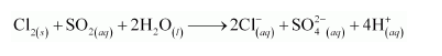# Chlorine is used to purify drinking water. Excess of chlorine is harmful.`
Question:

Chlorine is used to purify drinking water. Excess of chlorine is harmful. The excess of chlorine is removed by treating with sulphur dioxide. Present a balanced equation for this redox change taking place in water.

Solution:

The given redox reaction can be represented as: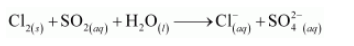The oxidation half reaction is: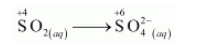The oxidation number is balanced by adding two electrons as: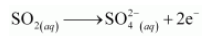The charge is balanced by adding 4H+ ions as: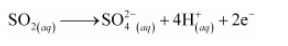The O atoms and H+ ions are balanced by adding 2H2O molecules as: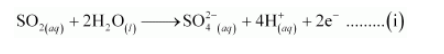The reduction half reaction is: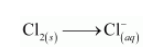The chlorine atoms are balanced as: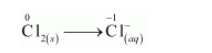The oxidation number is balanced by adding electrons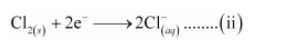The balanced chemical equation can be obtained by adding equation (i) and (ii) as: